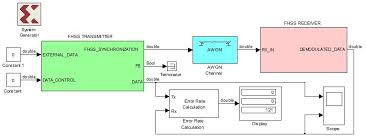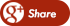﻿ what is AWGN Channal?Back

## what is AWGN Channal?

By Admin onAdditive  White Gaussian Noise

A basic and generally accepted model for thermal noise in communication channels, is the set of assumptions that

• the noise is additive, i.e., the received signal equals the transmit signal plus some noise, where the noise is statisticaly independent of the signal.
• the noise is white, i.e, the power spectral density is flat, so the autocorrelation of the noise in time domain is zero for any non-zero time offset.
• the noise samples have a Gaussian distribution.

Mostly it is also assumed that the channel is Linear and Time Invariant. The most basic results further asume that it is also frequency non-selective.

Optimal signal detection  in AWGN LTI channel

The theory for signal transmission over AWGN LTI channels is very well developed and covered in many excellent text books. Many fundamental theorems in signal detection theory have been deveoped during World War II, to improve and automate the radar detection of enemy airplanes and ships. The theory of the matched filter receiver is of particular interest. The concept was introduced by D.O. North with the RCA labs in Princeton, in 1943.). Figure: possible implementation of a matched filter receiver. The signal is multiplied by a locally stored reference copy and integrated over time (correlation

The matched filter correlates the incoming signal with a locally stored reference copy of the transmit waveform. The matched filter maximizes the signal-to-noise ratio for a known signal. It can be shown to be the optimal detector if

• the channel produces Additive White Gaussian Noise (AWGN),
• the channel is linear and time-invariant (LTI), and
• an exact time reference is available, the signal amplitude as a function of time is precisely known

For More Details Click Here